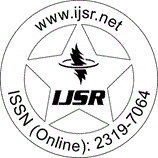International Journal of Science and Research (IJSR)
Call for Papers | Fully Refereed | Open Access | Double Blind Peer Reviewed

Research Paper | Electronics & Communication Engineering | Niger | Volume 11 Issue 9, September 2022

# Evaluation of Split-Step Fourier Transform for Tropospheric Electromagnetic Wave Propagation

Godwin Effiong  | Tebe Larry Ojukonsin  | Ayibapreye Kelvin Benjamin 

Abstract: In this letter, we adopt Kuttler and Dockery theoretical model using the split-step Fourier transform method which is a numerical solution of the parabolic solution to investigate the presence of rain drops in the troposphere. The parabolic equation (PE) model works by assuming that an outgoing EM wave dominates backscattered EM wave and factoring the operator in the frequency-domain wave equation to obtain an outgoing wave equation. Here, we study the interactions between propagating EM wave and rain drops in the troposphere. This procedure derives the scalar Helmholtz equation in spherical coordinates and uses conformal mapping to convert it to rectangular coordinate without requiring approximations. MATLAB programming software was used to simulate the numerical narrow angle split-step Fourier transform model and plots of field profile versus range and heights illustrate the effects of rain drops in the troposphere.

Keywords: Split step Fourier transform, Troposphere, Electromagnetic wave, Propagation

Edition: Volume 11 Issue 9, September 2022,

Pages: 522 - 527